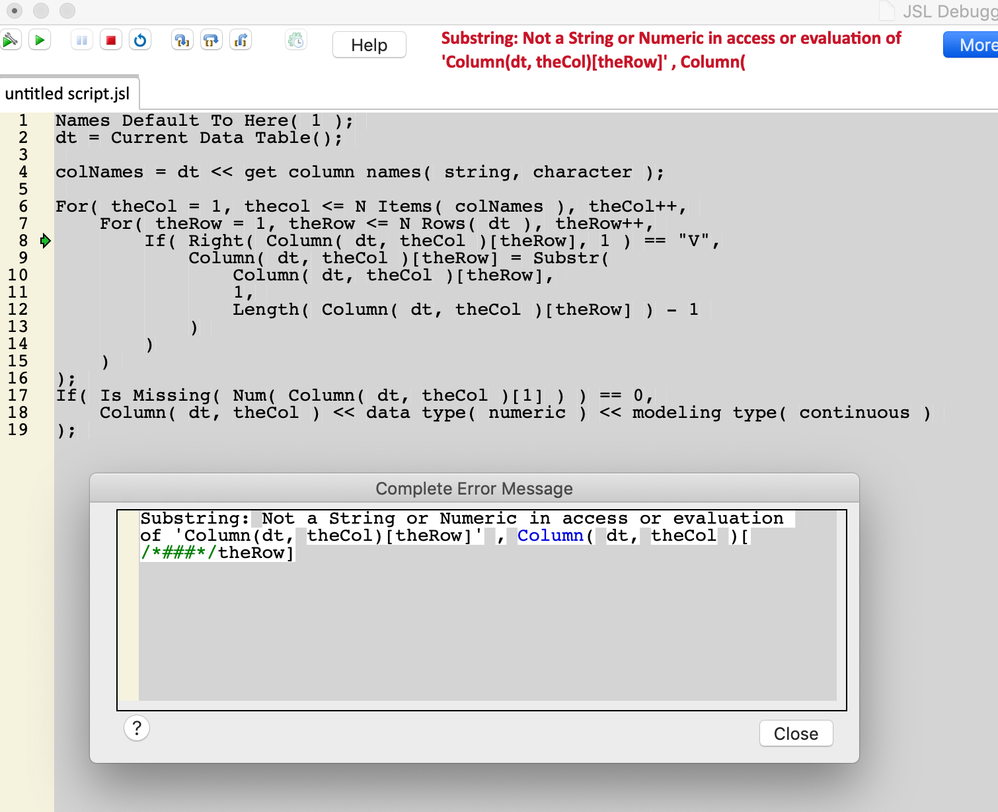Choose Language Hide Translation Bar
Highlighted

## How do I code find & replace in JSL, to remove text from a column & convert to number format.

Hello JMP community,

Once a week, I import .txt data with a battery voltage column (named "V1") where row values can be "3.45V", "3.34V", "3.12V", "3.23V", etc. I use Find & Replace manually to remove the letter "V" from the data, then manually change the data type from Character to Numeric. I suppose I don't quite understand how to include substitute() or mumger() in my scripts because I've tried & failed to create a working JSL for this simple task.

5 REPLIES 5
Highlighted

## Re: How do I code find & replace in JSL, to remove text from a column & convert to number format.

I've always been a big fan of producing the solution interactively first, then script. I know it is not always possible, but you can get close.

Opening the text file gives this script (for my example, of course) found in the Source script with the JMP table:

``````Open(
"C:\Testing.txt",
columns( New Column( "V1", Character, "Nominal" ) ),
Import Settings(
End Of Line( CRLF, CR, LF ),
End Of Field( Tab, Comma, CSV( 1 ) ),
Strip Quotes( 1 ),
Use Apostrophe as Quotation Mark( 0 ),
Use Regional Settings( 0 ),
Scan Whole File( 1 ),
Treat empty columns as numeric( 0 ),
CompressNumericColumns( 0 ),
CompressCharacterColumns( 0 ),
CompressAllowListCheck( 0 ),
Labels( 1 ),
Column Names Start( 1 ),
Data Starts( 2 ),
Year Rule( "20xx" )
)
)``````

You can modify as needed for your specific case.

Now for removing the V and converting to numeric, I would create a second column for that. This allows for error checking in case the voltage format changes, for some reason. Plus, it gives better data traceability. I created a column with this formula: Num( Word( 1, :V1, "V" ) ). I then looked at the "Copy Table Script" found in the table panel and pulled out the related part of the script to create the new column.

In JSL that would be:

``````New Column( "Numeric V1",
Numeric,
"Continuous",
Format( "Best", 12 ),
Formula( Num( Word( 1, :V1, "V" ) ) )
)``````

Putting the two together should be your script.

Dan Obermiller
Highlighted

## Re: How do I code find & replace in JSL, to remove text from a column & convert to number format.

Thanks, Dan,

I was able to fit this into my workflow as:

``````Names Default To Here( 1 );
:Name( "V1" ) << Set Name( "orig V1" );
New Column( "V1",
Numeric,
"Continuous",
Format( "Fixed Dec", 6, 2 ),
Formula( Num( Word( 1, :orig V1, "V" ) ) )
);``````

I appreciate the quick response!

Highlighted

## Re: How do I code find & replace in JSL, to remove text from a column & convert to number format.

See if something like this will work......it has not been fully tested, but it should be close to working

``````Names Default To Here( 1 );
dt = Current Data Table();

colNames = dt << get column names( string, character );

For( theCol = 1, thecol <= N Items( colNames ), theCol++,
For( theRow = 1, theRow <= N Rows( dt ), theRow++,
If( Right( Column( dt, theCol )[theRow], 1 ) == "V",
Column( dt, theCol )[theRow] = Substr(
Column( dt, theCol )[theRow],
1,
Length( Column( dt, theCol )[theRow] ) - 1
)
)
)
);
If( Is Missing( Num( Column( dt, theCol ) ) ) == 0,
Column( dt, theCol ) << data type( numeric ) << modeling type( continuous )
);``````
Jim
Highlighted

## Re: How do I code find & replace in JSL, to remove text from a column & convert to number format.

Hi Jim, your code didn't quite work, and I couldn't figure out why. I've uploaded a screenshot of JMP's debugger with the error message, and a subset of my data if you'd like to continue tackling this. I'd be very interested in this alternative solution!Highlighted

## Re: How do I code find & replace in JSL, to remove text from a column & convert to number format.

I did find a logic error in my code.  Below is an example data table with a small script that converts the values in place.

``````Names Default To Here( 1 );
dt = New Table( "Example",
New Column( "col1", character, formula( Char( Random Integer( 78, 100 ) ) || "V" ) ),
New Column( "col2", character, formula( Char( Random Integer( 78, 100 ) ) || "V" ) )
);
dt<<run formulas;
dt:col1 << delete property( "formula" );
dt:col2 << delete property( "formula" );

colNames = dt << get column names( string, character );

For( theCol = 1, thecol <= N Items( colNames ), theCol++,
For( theRow = 1, theRow <= N Rows( dt ), theRow++,
If( Right( Column( dt, theCol )[theRow], 1 ) == "V",
Column( dt, theCol )[theRow] = Substr(
Column( dt, theCol )[theRow],
1,
Length( Column( dt, theCol )[theRow] ) - 1
)
)
);

If( Is Missing( Num( Column( dt, theCol ) ) ) == 0,
Column( dt, theCol ) << data type( numeric ) << modeling type( continuous )
);
);``````
Jim
Article Labels

There are no labels assigned to this post.